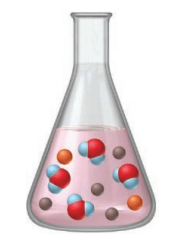# Problem: The flask represents the products of the titration of 25 mL of sulfuric acid with 25 mL of sodium hydroxide.Write balanced molecular, total ionic, and net ionic equations for the reaction.

###### FREE Expert Solution

For this problem, we are being asked to write balanced molecular, total ionic, and net ionic equations for the reaction.

We’re going to determine the spectator ions using the following steps:

Step 1. Write the overall balanced reaction
Step 2. Write the total or full ionic equation
Step 3. Identify the spectator ions

Step 1. Write the overall balanced reaction

Reactants:

Sulfuric acid → H2SO4(aq) → aqueous → will break up into its ions

▪ H → Group 1A → H+
▪ SO4 → polyatomic anion → SO42-

92% (70 ratings)###### Problem Details

The flask represents the products of the titration of 25 mL of sulfuric acid with 25 mL of sodium hydroxide.Write balanced molecular, total ionic, and net ionic equations for the reaction.

Frequently Asked Questions

What scientific concept do you need to know in order to solve this problem?

Our tutors have indicated that to solve this problem you will need to apply the Complete Ionic Equations concept. You can view video lessons to learn Complete Ionic Equations. Or if you need more Complete Ionic Equations practice, you can also practice Complete Ionic Equations practice problems.

What professor is this problem relevant for?

Based on our data, we think this problem is relevant for Professor Halpin's class at NYU.

What textbook is this problem found in?

Our data indicates that this problem or a close variation was asked in Chemistry: The Molecular Nature of Matter and Change - Silberberg 8th Edition. You can also practice Chemistry: The Molecular Nature of Matter and Change - Silberberg 8th Edition practice problems.